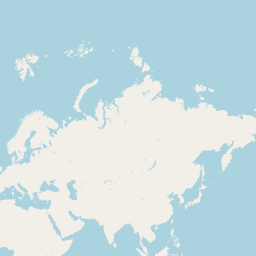# Mapserver tiles - trouble with projection

I'm trying to render tiles with shp2img tool from Mapserver suite and my tiles aren't matching. Tiles are numbered like in openstreetmaps.org. This is an attempt to draw tile (level 1, x=1, y=0) which has extent [xmin=0, ymin=0, xmax=180, ymax=90]:
http://img69.imageshack.us/img69/4112/z0x1y0.png
This picture is rendered with extent [xmin=0, ymin=0, xmax=180, ymax=90]. See the problem? Ymin is clearly not zero here.
And this one with extent [xmin=0, ymin=0, xmax=90, ymax=90]:
http://img834.imageshack.us/img834/5489/z0x1y0v2.png
Now ymin looks zero as it should. It seems like Mapserver tries to keep aspect ratio and is extending rendered area to do that. But this ruins my tile service.
How do I setup projection in my MAP file to get matching tiles? Or what else to do to get tiles right.

Update: this is how tile z=1, x=1, y=0 looks in openstreetmaps.org, I guess I need reproject my map in such projection?Some details:
ogrinfo output:

``````Layer SRS WKT:
GEOGCS["Unknown",
DATUM["MIF 0",
SPHEROID["WGS_84_MAPINFO_Datum_0",6378137.01,298.257223563]],
PRIMEM["Greenwich",0],
UNIT["Degree",0.017453292519943295]]
``````

Projection section from MAP file:

``````PROJECTION
"init=epsg:4326"
END
``````

Resolved, I just set projection in MAP file to Mercator:

``````PROJECTION
"proj=merc"
"a=6378137"
"b=6378137"
"lat_ts=0.0"
"lon_0=0.0"
"x_0=0.0"
"y_0=0"
"k=1.0"
"units=m"
``````PROJECTION